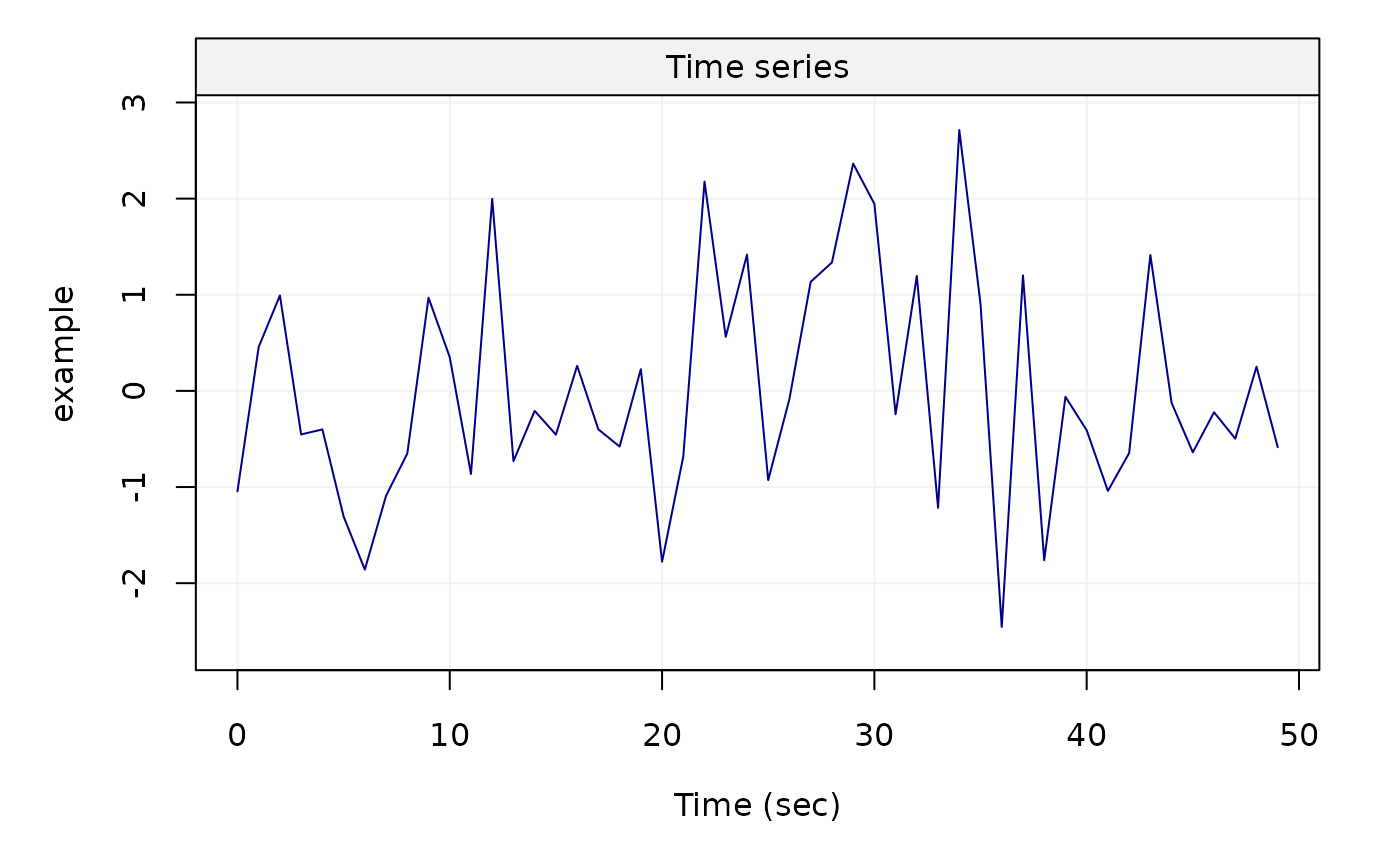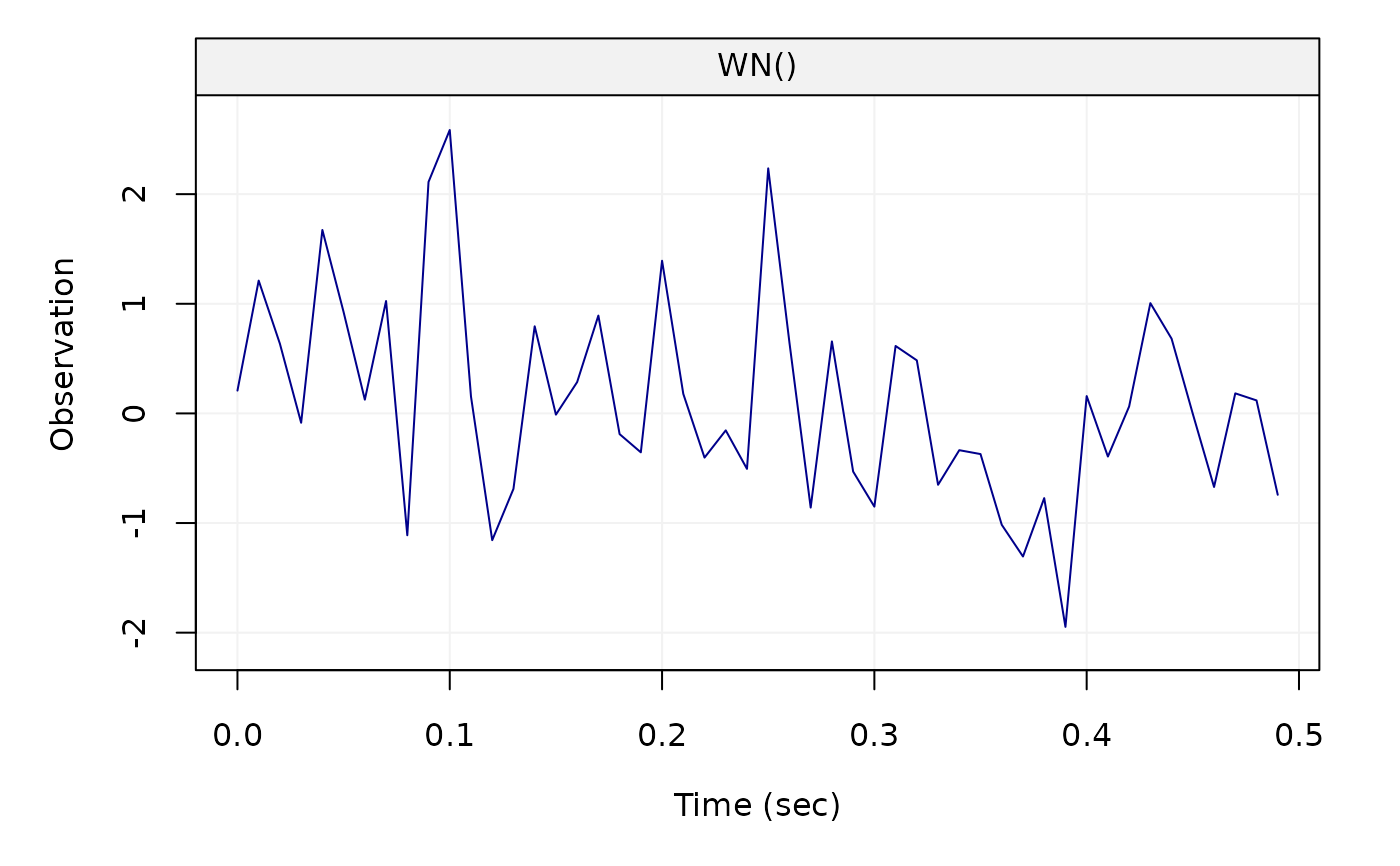Takes a time series and turns it into a time series oriented object that can be used for summary and graphing functions in the simts package.

gts(
data,
start = 0,
end = NULL,
freq = 1,
unit_ts = NULL,
unit_time = NULL,
name_ts = NULL,
name_time = NULL,
data_name = NULL,
Time = NULL,
time_format = NULL
)

## Arguments

data

A one-column matrix, data.frame, or a numeric vector.

start

A numeric that provides the time of the first observation.

end

A numeric that provides the time of the last observation.

freq

A numeric that provides the rate/frequency at which the time series is sampled. The default value is 1.

unit_ts

A string that contains the unit of measure of the time series. The default value is NULL.

unit_time

A string that contains the unit of measure of the time. The default value is NULL.

name_ts

A string that provides an identifier for the time series data. Default value is NULL.

name_time

A string that provides an identifier for the time. Default value is NULL.

data_name

A string that contains the name of the time series data.

Time

A numeric or character vector containing the times of observations. Default value is NULL. See x object in as.Date function.

time_format

A string specifiying the format of 'Time'. If not provided, 'Time' is assumed to be all integers. Default value is NULL. See format argument in as.Date function.

## Value

A gts object

## Author

James Balamuta and Wenchao Yang

## Examples

m = data.frame(rnorm(50))
x = gts(m, unit_time = 'sec', name_ts = 'example')
plot(x)x = gen_gts(50, WN(sigma2 = 1))
x = gts(x, freq = 100, unit_time = 'sec')
plot(x)